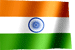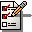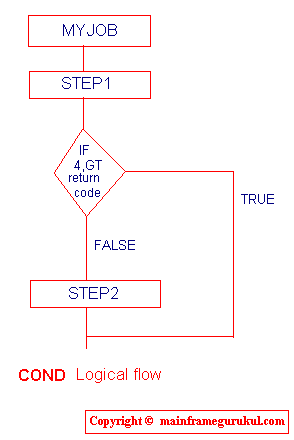RAMESH KRISHNA REDDYOnline Tutorials | PREV | TOP | NEXT

DRONA SERIES
JCL STUDY MATERIAL

CHAPTER - 5

 ``` PARAMETERS IN BOTH STATEMENTS (JOB and EXEC) COND REGION TIME These parameters can code in both JOB and EXEC statements, When we code these parameters on both statement, JOB - REGION will override EXEC - REGION JOB - COND will override EXEC - COND EXEC - TIME will overrirde JOB - TIME Now let us see details of these parameters REGION - Specifies the amount of space should be used at the time of running a job/step Syntax -> REGION=< value >K or REGION=< value >M Example JCL -> //MYJOB JOB (AE32),'RAMESH' //STEP1 EXEC PGM=COBPROG, // REGION=67KAll available storage is assigned to the job or jobstep, If REGION is coded 0K or 0M COND - Each step sends an a return code to the system upon completion. This is called condition code. COND parameter is used to control the execution of subsequent job steps, depending on the condition code that is returned for prior step. Return code number between 0 and 4095 Syntax -> COND=(comparsion-code,condition) (If coded on job) COND=(comparsion-code,condition [,stepname] [,EVEN / ONLY]) (If coded on step) condition can be GT,GE,LT,LE,EQ,NE Example JCL -> COND coded on JOB //MYJOB JOB (R475),'KRISHNA REDDY' // COND=(4,GT) //STEP1 EXEC PGM=COBPROG //STEP2 EXEC PGM=COBPROG2 In this example we are specifing that if the number 4 is grater than the return code of STEP1, then STEP2 is to be bypassed. It is elaborated further in the following imageCOND coded on EXEC statement //MYJOB JOB (U769),'KRISHNA REDDY' //STEP1 EXEC PGM=PROG1 //STEP2 EXEC PGM=COBPROG, // COND=(8,EQ,STEP1) // In this example the COND parameter is used to specify that STEP2 should be by passed if 8 is equal to the return code issued by STEP1. TIME - The time parameter is used to specify the amount of CPU time that a job or job step is permitted to utilize. Syntax -> TIME=minutes OR TIME=([minutes] [,seconds]) minutes can be between 1 and 1439 seconds can be between 1 and 59 Example JCL -> If coded on JOB //MYJOB JOB (E234),'RAMESH KRISHNA',TIME=(20,30) //STEP1 EXEC PGM=COBPROG1 //STEP2 EXEC PGM=COBPROG2 //STEP3 EXEC PGM=COBPROG3 In this example 20 minutes 30 seconds time alloted to job. All steps in this job STEP1 STEP2 STEP3 should complete its task within 20 min. 30 sec. If coded on STEP //MYJOB JOB (R567),'KRISHNA' //STEP1 EXEC PGM=COBPRO // TIME=30 In this example 30 min. time is alloted to STEP1. If STEP1 requires more than 30 min. MYJOB will terminate abnormally. If coded on both STEP AND JOB //SECOND JOB ,'R. KRISHNA',TIME=3 //STEP1 EXEC PGM=C,TIME=2 //STEP2 EXEC PGM=D,TIME=2 In this example, the job is allowed 3 minutes of execution time. Each step is allowed 2 minutes of execution time. Should either step try to execute beyond 2 minutes, the job will terminate beginning with that step. If STEP1 executes in 1.74 minutes and if STEP2 tries to execute beyond 1.26 minutes, the job will be terminated because of the 3-minute time limit specified on the JOB statement.By coding TIME=1440 or TIME=NOLIMIT, It will give a job or step an unlimited amount of time.To allow a job or step to use the maximum amount of time, code TIME=MAXIMUM. Coding TIME=MAXIMUM allows the job or step to run for 357912 minutes. (Approx. 248 days) ```

NEXT CHAPTER TOPIC : DD STATEMENT

Visit jcl books section in this site for good books